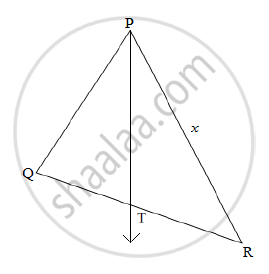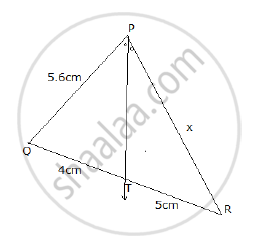# In the figure given below, Ray PT is bisector of ∠QPR. If PQ = 5.6 cm, QT = 4 cm and TR = 5 cm, find the value of x . - Geometry

In the figure given below, Ray PT is bisector of ∠QPR. If PQ = 5.6 cm, QT = 4 cm and TR = 5 cm, find the value of x .#### Solution

Given: ray PT is bisector of ∠QPR.

PQ = 5.6 cm, QT = 4 cm and TR = 5 cm.

In a triangle, the angle bisector divides the side opposite to the angle in the ratio of the remaining sides.

"QT"/"TR"="PQ"/"PR"

4/5=5.6/x

x=(5.6xx5)/4

x=28/4=7

PR=7cmConcept: Similarity of Triangles
Is there an error in this question or solution?